In this post I will discuss bifurcation.

“In general, a small variation in some parameter produces small, continuous changes in the system output, so that the system is said to be structurally stable. However, for some specific parameter values, a small variation can induce a strong qualitivative change in the solution. Such a behavior is called a bifurcation, and the system is said to be structurally unstable for these parameter values.”

Consider the ordinary differential equation:

``````x_t  = r x - x^3
``````

where `r` is the parameter of interest, subscript `t` is the time derivative of `x`. An equilibrium of the system is a point where the system remains unchanged or equivalently

``````x_t = 0
``````

In our case if `r` is greater than zero then there are three equilibriums:

``````x = 0, -root(r), + root(r)
``````

We can plot the derivative `x_t` against `x`: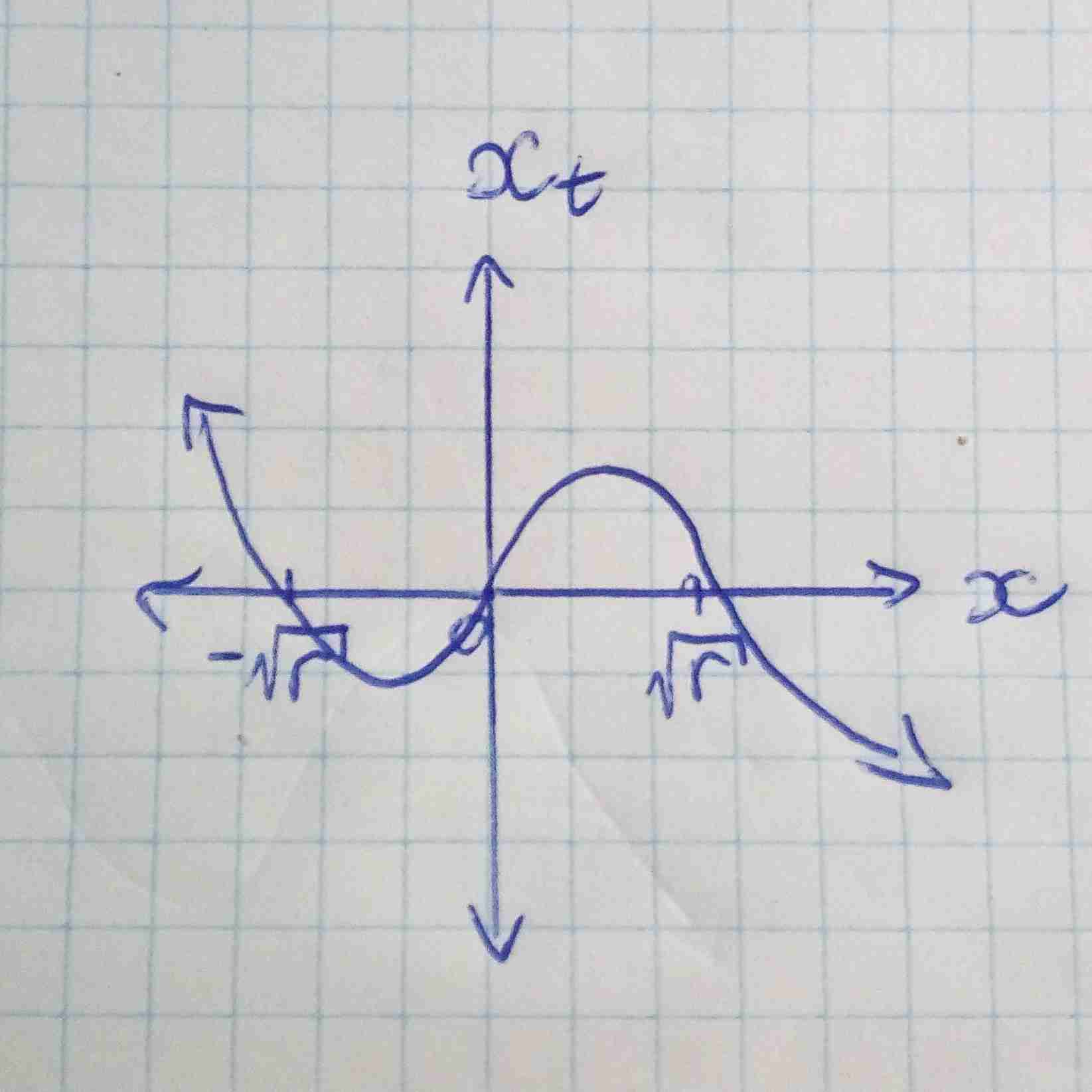The first equilibrium is unstable since a slight perturbation will cause to move away from it (similar to a ball on top of a hill being slightly pushed will roll away). It is unstable because if `x` is slightly less than zero then the negative derivative (refer to previous plot) will cause `x` to decrease away from 0 and similarly if `x` is slightly greater than zero then the positive derivative will cause `x` to increase away from 0. Using similar logic the other two equilbiriums can be shown to be stable (a ball in a valley pushed will roll back).

When `r` is less than or equal to zero there is only one equilibrium (`x=0` which is stable). Once again we plot `x_t` against `x`: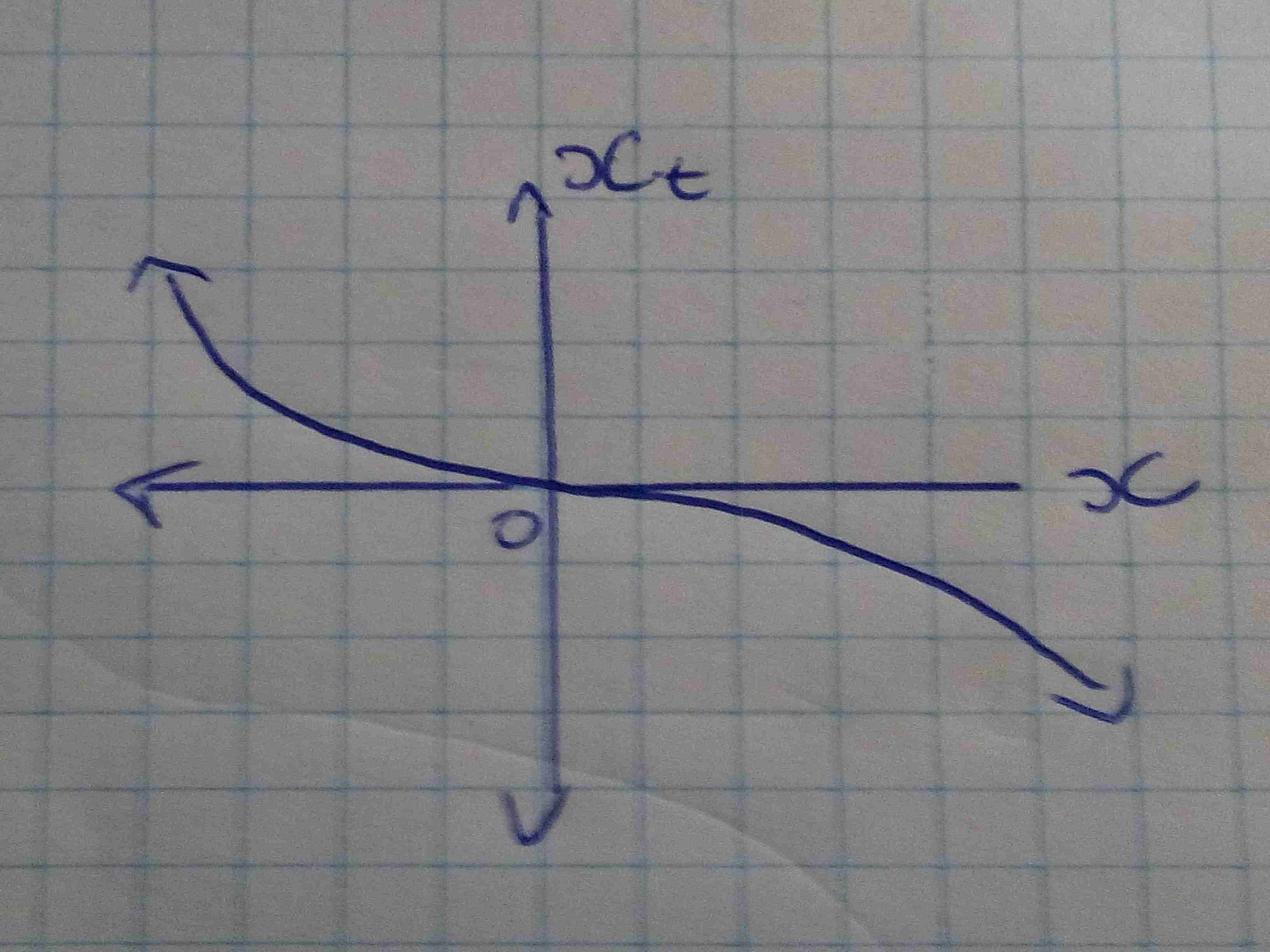When varying `r` from negative to positive we move from one stable point to two stable points. `r=0` is the bifurcation point where small variation will cause strong qualititative change in the solution.

Now let’s consider a more interesting system - the logistic map equation:

``````x_{t+1} = r x_{t} (1-x_{t})
``````

where `r` is the parameter. We will restrict `r` to be in the range `0` to `4` (this provides that if `x_{n}` is between 0 and 1 then `x_{n+1}` will also be between 0 and 1 - can be checked via derivative tests). The logistic map reprents a population model where `x_{n}` between `0` and `1` represents the percentage out of a total maximum possible population. Below are some sample populations for different `r` values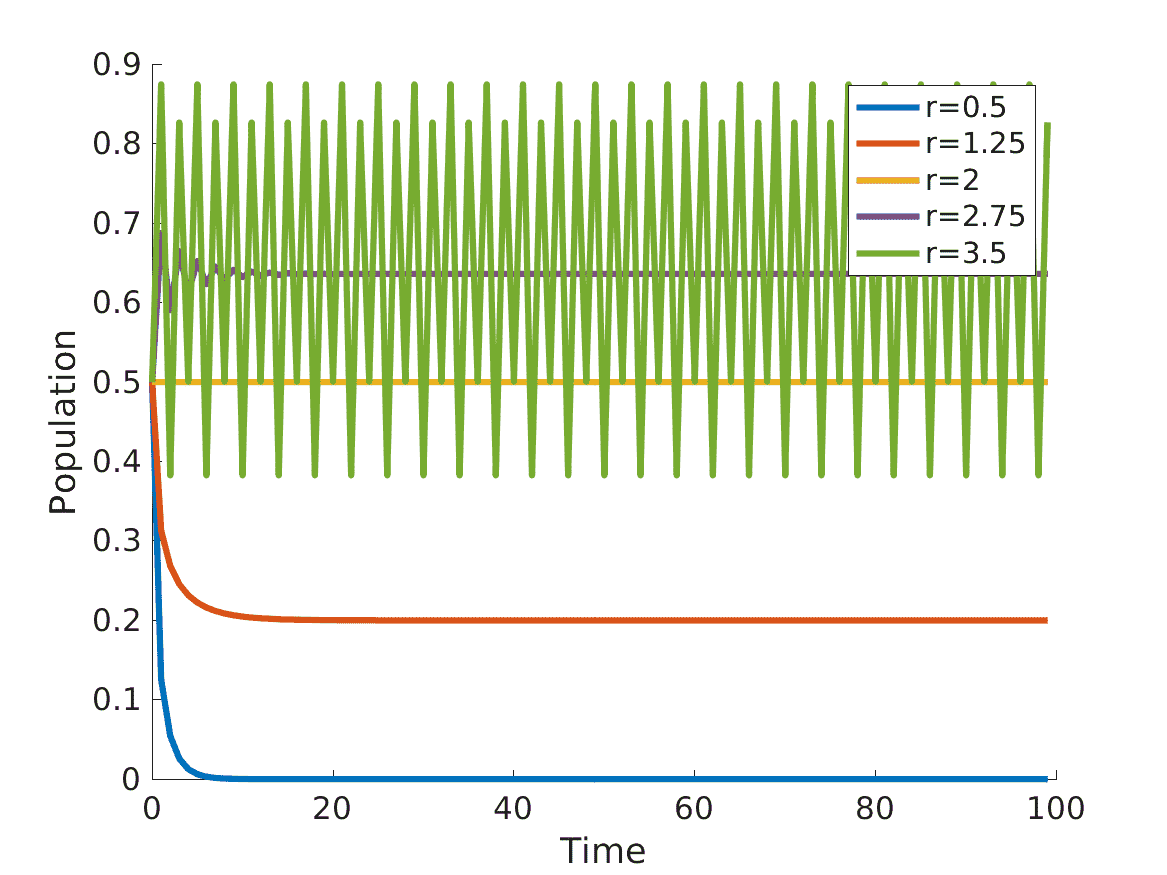For `r=0.5` the steady value is `0` meaning that the population starting at `x_{0} = 0.5` will lead to population extinction (`x_{n} = 0`). For `r=1.25` the population reaches steady state of about `0.2`. `r=2.75` and `r=2` also reach a single steady state. Now for `r=3.5` things get interesting - there are now `4` steady values about which the curve oscillates about regularly: `0.8269, 0.5009, 0.8750, 0.3828`. By changing the parameter `r` from `2.75` to `3.5` we have change the amount of steady values from `1` to `4`. This leads us to plot a bifurcation diagram. Below the diagram plots for various `r` (Growth Rate) the steady values. For `r` less than `3` there is only one steady value and at about `3` (bifurcation point) we now have two steady points and once `r` is about `3.5` there are four (as demonstrated earlier).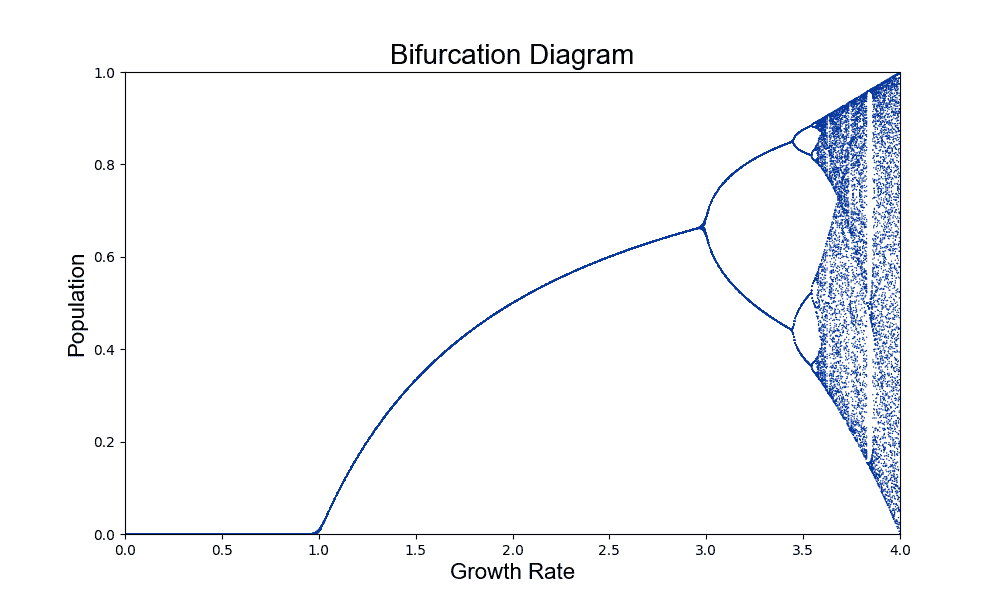If we zoom in to a smaller range we see the following: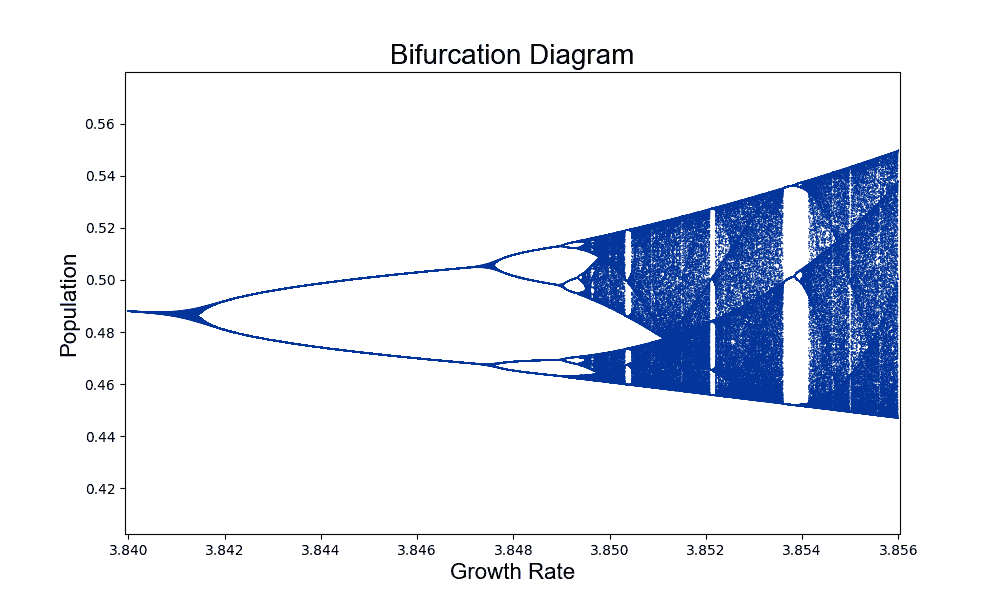and if we zoom in even further we start noticing a fractal type pattern emerging - same structure at every scale. From the simple logistic map equation we obtain a beautiful pattern.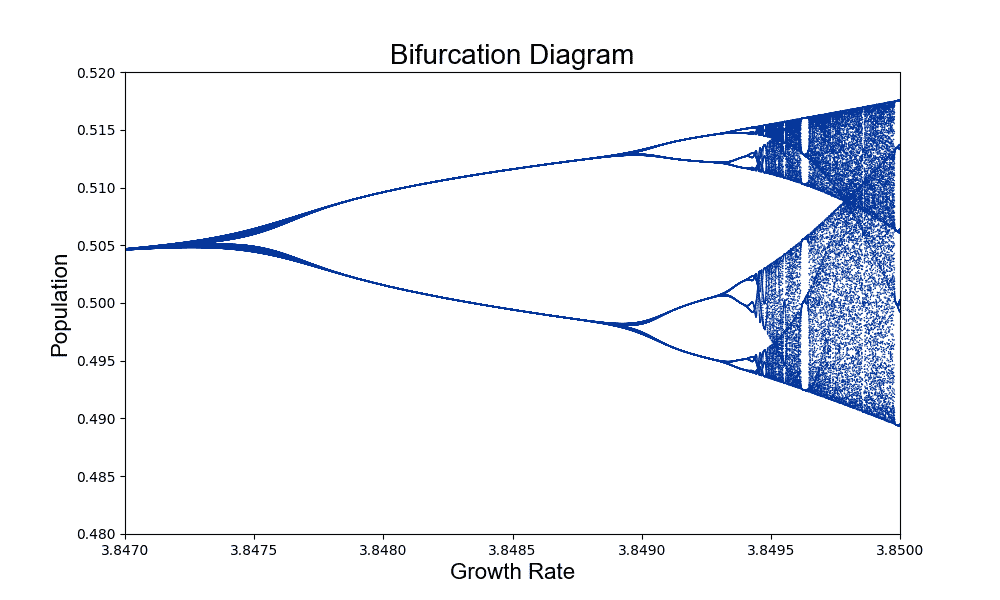Some of the code used to generate the figures, insipiration as well as additional reading material can be found here:

1. Boccaletti, Stefano, et al. Synchronization: From Coupled Systems to Complex Networks. Cambridge University Press, 2018
2. Boeing, Geoff. “Visual analysis of nonlinear dynamical systems: chaos, fractals, self-similarity and the limits of prediction.” Systems 4.4 (2016): 37.
3. https://www.math.ucdavis.edu/~hunter/m207/m207.pdf
4. https://github.com/gboeing/pynamical